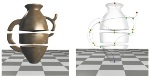## Computing Reeb graphs as a union of contour trees.Harish Doraiswamy and Vijay Natarajan.
IEEE Transactions on Visualization and Computer Graphics, 19 (2), 2013, 249-262.

### Abstract

The Reeb graph of a scalar function tracks the evolution of the topology of its level sets. This paper describes a fast algorithm to compute the Reeb graph of a piecewise linear function defined over manifolds and non-manifolds. The key idea in the proposed approach is to maximally leverage the efficient contour tree algorithm to compute the Reeb graph. The algorithm proceeds by dividing the input into a set of subvolumes that have loop-free Reeb graphs using the join tree of the scalar function and computes the Reeb graph by combining the contour trees of all the subvolumes. Since the key ingredient of this method is a series of union-find operations, the algorithm is fast in practice. Experimental results demonstrate that it outperforms current generic algorithms by a factor of up to two orders of magnitude, and has a performance on par with algorithms that are catered to restricted classes of input. The algorithm also extends to handle large data that do not fit in memory.